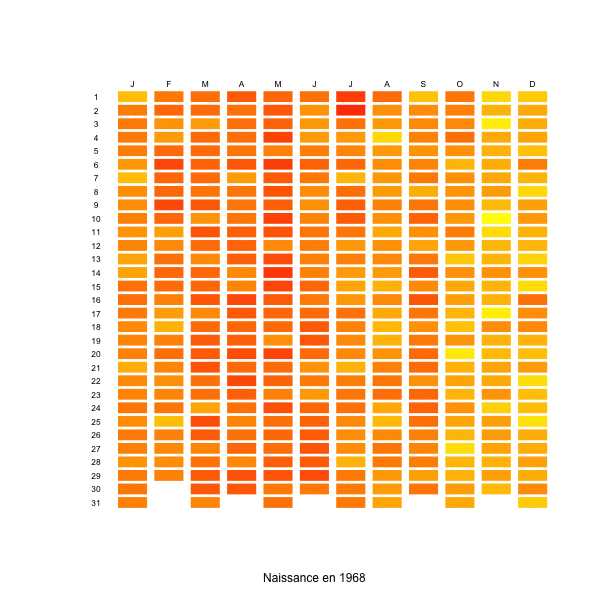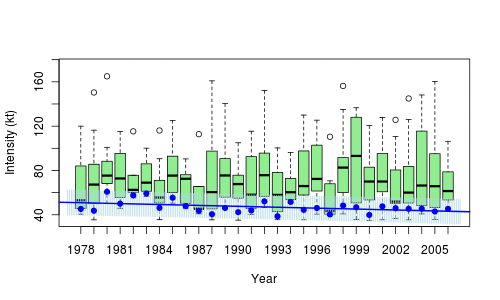# Proportion of people alive in 1945 that are still alive

In demography, we like to use life tables to estimate the probability that someone born in 1945 (say) is still alive nowadays.  But another interesting quantity might be the probability that someone alive in 1945 is still alive nowadays.

The main difference is that we do not know when that person, alive in 1945, was born. Someone who was old in 1945 is very unlikely still alive in 2017. To compute those probabilities, we can use datasets from http://www.mortality.org/hmd/. More precisely, we need both death and birth data. I assume that datasets (text files) were downloaded (it is necessary to register – for free – to get the data).

```D=read.table("FRDeaths_1x1.txt",skip=1,header=TRUE) B=read.table("FRBirths.txt",skip=1,header=TRUE)```

In the death dataset, there is a “110+” for people older than 110 years. For convenience, let us cap our observations at 110 years old,

```D\$Age=as.numeric(as.character(D\$Age)) D\$Age[is.na(D\$Age)]=110```

Consider now a first function that will return, for people born in 1930 (say) two informations

• the number of people (here, let us consider women only) born in 1930 (from the birth database)
• the number of death of people of age 0 in 1930, people of age 1 in 1931, people of age 2 in 1932, etc…

The code is simple

```nb=function(y=1930){ debut=1816 MatDFemale=matrix(D\$Female,nrow=111) colnames(MatDFemale)=debut+0:198 cly=y-debut+1:111 deces=diag(MatDFemale[,cly[cly%in%1:199]]) return(c(B\$Female[B\$Year==y],deces))}```

We have a single number for the number of births, and then a vector for the number of deaths. Consider now another function. Consider the people born in 1930. We want to get two numbers : the number of people still alive in 1945 (say), and the number of people still alive nowadays. The ratio will be the proportion of people born in 1930 that were alive in 1945, that are still alive in 2015.

```pop=function(ne=1930,an=1945){ comptage=nb(ne) s=0 if(an>ne) s=sum(comptage[seq(2,1+an-ne)]) p1=max(comptage-s,0) p2=max(p1-sum(comptage[seq(2+an-ne,length(comptage))]),0) c(p1,p2) }```

Then, for a given year (say 1945), to get the proportion of people alive in 1945 that are still alive today, we need to count how many people born in 1944 were still alive in 1945, and in 2015, but also born in 1943, 1942, etc, And we simply consider the ratio of the total number of people alive in 2015 over the total number of people alive in 1945

```ptn=function(y=1945){ V=Vectorize(function(x) pop(x,y))(1816:y) sum(V[2,!is.na(V[2,])])/sum(V[1,!is.na(V[1,])]) }```

Hence, 22% of those alive in 1945 are still alive in 2015,

```> ptn(1945)  0.2209435```

Actually, instead of looking only at 1945, it is possible to get a plot

```P=Vectorize(ptn)(1900:2010) plot(1900:2010,P,type="l",ylim=0:1)```For instance,

```> ptn(1975)  0.6377413```

i.e. 63.7% of those alive in 1975 are stil alive 40 years after. That is a rather interesting function, I was surprised that I couldn’t find it is standard demographical R package…

# Births and week-ends, in France

This week, I have seen on the internet (sorry, I cannot find proper references) the graph produced here on the right: which birthday is most likely ? The fact that I have no further information is important, since I do not know in which country such a graph was obtained. At least, I know it should not be France…

In France, I have already mentioned that there is a strong week-end effect: nowadays, there is 25% less deliveries during week-ends than during the week. Calot (1981) observed already that there were less deliveries on Sundays. This has been confirmed more recently, e.g. in http://www.lepoint.fr/ or http://www.prepabl.fr/, with a significant difference between week days, and week-ends. Here  is the number of birth per day, over 40 years, with in blue the average trend during the week, and in red, during week-ends,

```naissance=read.table(
"http://freakonometrics.free.fr/naissanceFR2.txt")
attach(naissance)
date=as.Date(date)
plot(date, nbre,cex=.5)
t2=as.POSIXlt(date)
jour=t2\$wday
X=naissance\$date
Y=naissance\$nbre
J=jour
df=data.frame(X,Y,J)
library(splines)
regs=lm(Y~bs(X,df=20),data=df[jour%in%c(0,6),])
Yp=predict(regs,newdata=df)
lines(X,Yp,col="red",lwd=3)
regs=lm(Y~bs(X,df=20),data=df[jour%in%1:5,])
Yp=predict(regs,newdata=df)
lines(X,Yp,col="blue",lwd=3)```If we look at the evolution of the ratio week-ends over weeks days, we have the following graph

```t2=as.POSIXlt(date)
jour=t2\$wday
jour=jour[1:(1982*7)]
nbre2=jour
for(i in 1:1982){
taux=sum(nbre[6:7+7*(i-1)])/
sum(nbre[1:5+7*(i-1)])/2*5
nbre2[1:5+7*(i-1)]=nbre[1:5+7*(i-1)]*taux
nbre2[6:7+7*(i-1)]=nbre[6:7+7*(i-1)]
nbre2[1:7+7*(i-1)]=
mean(nbre[1:7+7*(i-1)])/mean(nbre2[1:7+7*(i-1)])*
nbre2[1:7+7*(i-1)]
}
nbretaux=jour
for(i in 1:1982){
taux=sum(nbre[6:7+7*(i-1)])/
sum(nbre[1:5+7*(i-1)])/2*5
nbretaux[1:7+7*(i-1)]=taux
}
plot(date[1:length(nbre2)],nbretaux)
X= date[1:length(nbre2)]
Y=nbretaux
library(splines)
reg=lm(Y~bs(X,df=20))
Yp=predict(reg)
lines(X,Yp,col="red",lwd=3)```In the beginning of the 70’s, during week-ends, there were 5% less deliveries, but 25% less around 2000. It is then possible to produce the same kind of graphs as the one above, per year of birth. And here, we clearly observe the importance of the week end effect (maybe also because of color choice)

```naissance=read.csv(
"http://freakonometrics.free.fr/naissanceFR.csv",
sep=";")
M=as.matrix(naissance[,3:ncol(naissance)])
BIRTH=as.vector(t(M))
YEAR=rep(1968:2005,each=12*31)
MONTH=rep(rep(1:12,each=31),38)
DAY=rep(1:31,12*38)
X=NA
for(y in 1968:2005){
sbase=base[YEAR==y,]
X=c(X,sbase\$BIRTH/sum(sbase\$BIRTH,
na.rm=TRUE))
}
base=data.frame(YEAR,MONTH,DAY,
BIRTH,BIRTHDAYPROB=X[-1])

m1=min(base\$BIRTHDAYPROB,na.rm=TRUE)
m2=max(base\$BIRTHDAYPROB,na.rm=TRUE)
y=1980
colr=rev(heat.colors(100))
sbase=base[YEAR==y,]
plot(0:1,0:1,col="white",xlim=c(-1,12),
ylim=c(-31,1),axes=FALSE,xlab=
paste("Naissance en",y,sep=" "),ylab="")
for(x in 1:nrow(sbase)){
a=sbase\$MONTH[x];b=sbase\$DAY[x]
polygon(c(a-.9,a-.9,a-.1,a-.1),-c(b-.9,b-.1,
b-.1,b-.9),col=colr[(sbase\$BIRTHDAYPROB[x]-m1)/
(m2-m1)*100],border=NA)
}
text((1:12)-.5,.5,c("J","F","M","A","M","J","J",
"A","S","O","N","D"),cex=.7)
text(-.5,-(1:31)+.5,1:31,cex=.7)```# Talk on quantiles at the R Montreal groupThis afternoon, I will be giving a two-hour talk at McGill on quantiles, quantile regressions, confidence regions, bagplots and outliers. Before defining (properly) quantile regressions, we will mention regression on (local) quantiles, as on the graph below, on hurricanes,In order to illustrate quantile regression, consider the following natality database,

```base=read.table(
"http://freakonometrics.free.fr/natality2005.txt",

We can use it produce those nice graphs we can find in several papers, modeling weight of newborns,

```u=seq(.05,.95,by=.01)
coefstd=function(u) summary(rq(WEIGHT~SEX+
SMOKER+WEIGHTGAIN+BIRTHRECORD+AGE+ BLACKM+
BLACKF+COLLEGE,data=base,tau=u))\$coefficients[,2]
coefest=function(u) summary(rq(WEIGHT~SEX+
SMOKER+WEIGHTGAIN+BIRTHRECORD+AGE+ BLACKM+
BLACKF+COLLEGE,data=base,tau=u))\$coefficients[,1]
CS=Vectorize(coefstd)(u)
CE=Vectorize(coefest)(u)```The slides can be downloaded on the blog, as well as the R-code.